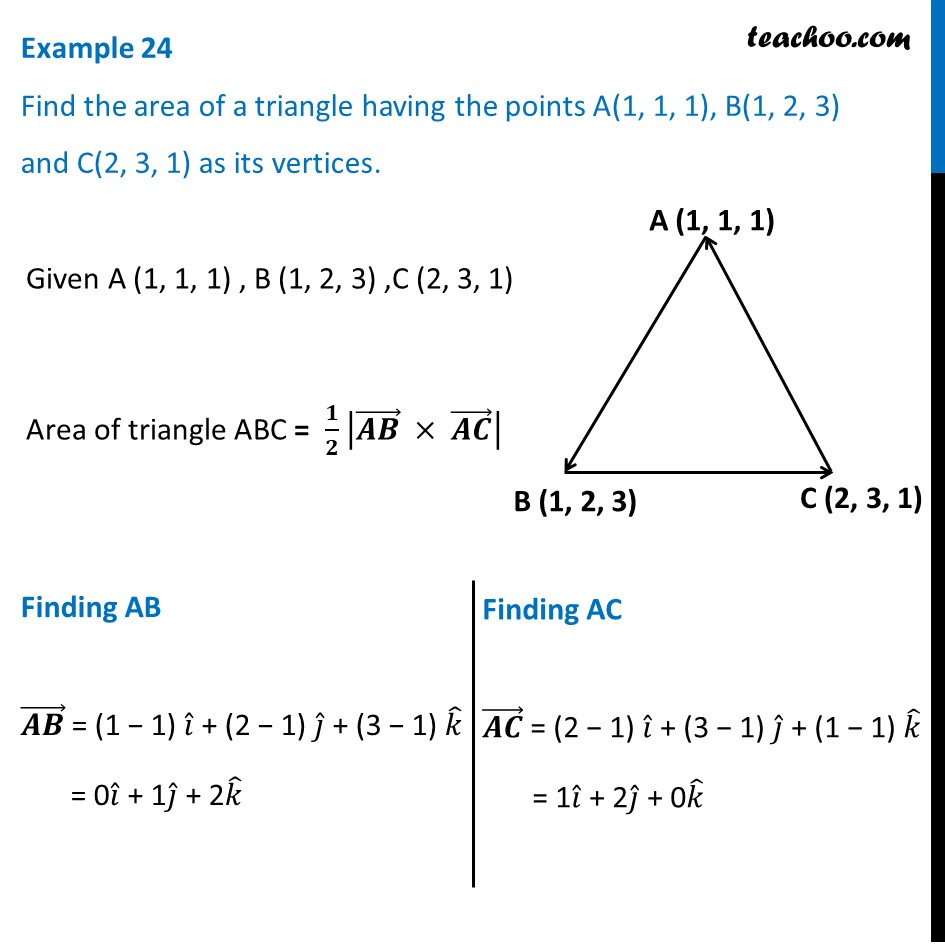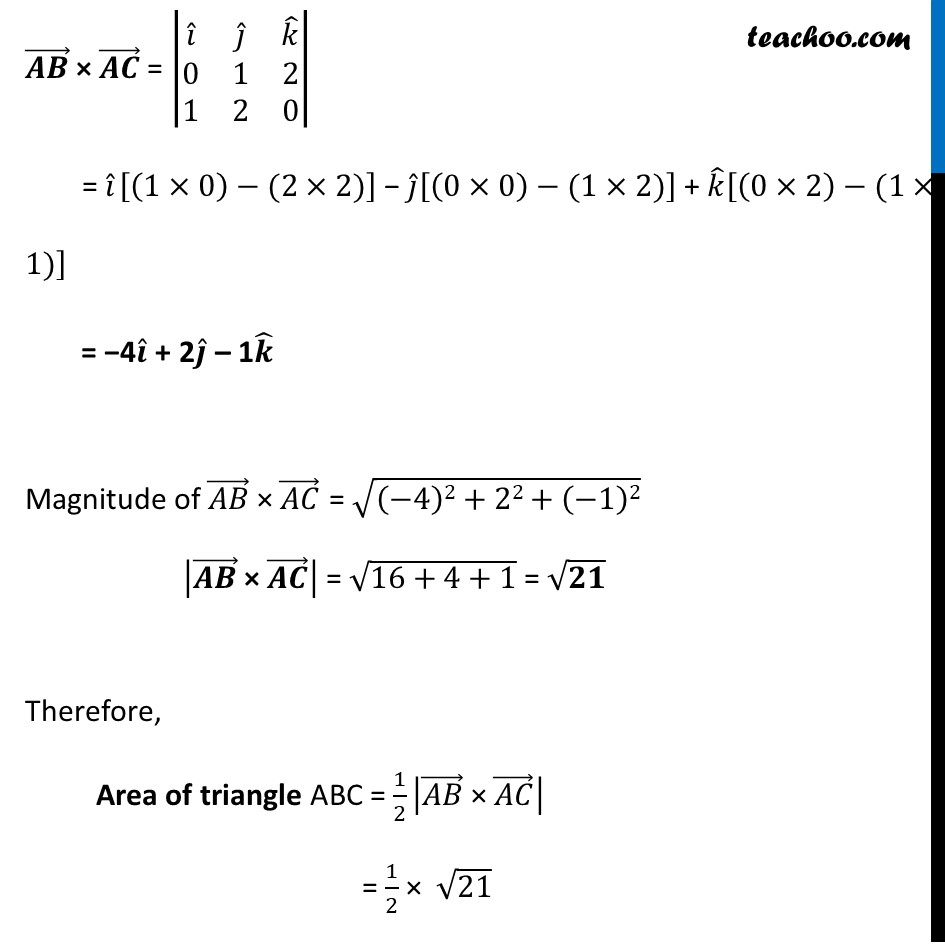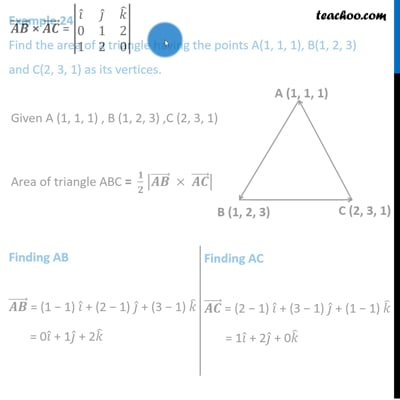Vector product - Area

Chapter 10 Class 12 Vector Algebra
Concept wiseThis video is only available for Teachoo black users

Introducing your new favourite teacher - Teachoo Black, at only ₹83 per month

### Transcript

Example 24 Find the area of a triangle having the points A(1, 1, 1), B(1, 2, 3) and C(2, 3, 1) as its vertices. Given A (1, 1, 1) , B (1, 2, 3) ,C (2, 3, 1) Area of triangle ABC = 𝟏/𝟐 |(𝑨𝑩) ⃗ × (𝑨𝑪) ⃗ | Finding AB (𝑨𝑩) ⃗ = (1 − 1) 𝑖 ̂ + (2 − 1) 𝑗 ̂ + (3 − 1) 𝑘 ̂ = 0𝑖 ̂ + 1𝑗 ̂ + 2𝑘 ̂ Finding AC (𝑨𝑪) ⃗ = (2 − 1) 𝑖 ̂ + (3 − 1) 𝑗 ̂ + (1 − 1) 𝑘 ̂ = 1𝑖 ̂ + 2𝑗 ̂ + 0𝑘 ̂ (𝑨𝑩) ⃗ × (𝑨𝑪) ⃗ = |■8(𝑖 ̂&𝑗 ̂&𝑘 ̂@0&1&2@1&2&0)| = 𝑖 ̂ [(1×0)−(2×2)] − 𝑗 ̂[(0×0)−(1×2)] + 𝑘 ̂[(0×2)−(1×1)] = −4𝒊 ̂ + 2𝒋 ̂ – 1𝒌 ̂ Magnitude of (𝐴𝐵) ⃗ × (𝐴𝐶) ⃗ = √((−4)2+22+(−1)2) |(𝑨𝑩) ⃗" × " (𝑨𝑪) ⃗ | = √(16+4+1) = √𝟐𝟏 Therefore, Area of triangle ABC = 1/2 |(𝐴𝐵) ⃗" × " (𝐴𝐶) ⃗ | = 1/2 × √21 = √𝟐𝟏/𝟐